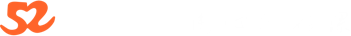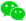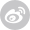运用特有的画线函数画出波段高低点线段，直观显示K线的形态结构便于投资分析决策。运用独特的函数算法，求出波段高低点的值进行排列对比，从而实现各种形态选股条件。

1买:

{底背离,柱背离}

DIFF:=EMA(CLOSE,12) - EMA(CLOSE,26);

DEA:=EMA(DIFF,9);

MACD:=2*(DIFF-DEA);

QZQ:=BARSLAST(REF(MACD,1)<0 AND MACD>0);

QM:=LLVBARS(MACD,QZQ+20);

MQDZ:=IF(QM>QZQ,REF(MACD,QM),0);

XG1:=MACD<0 AND MACD>MQDZ

AND CLOSE=LLV(CLOSE,QZQ+20) AND MQDZ<0;

XG:REF(XG1,1)=1 AND MACD>REF(MACD,1);

{双底背离,柱加线}

DIFF:=EMA(CLOSE,12) - EMA(CLOSE,26);

DEA:=EMA(DIFF,9);

MACD:=2*(DIFF-DEA);

QZQ:=BARSLAST(REF(MACD,1)<0 AND MACD>0);

QM:=LLVBARS(MACD,QZQ+20);

MQDZ:=IF(QM>QZQ,REF(MACD,QM),0);

QF:=LLVBARS(DIFF,QZQ+20);

DQDZ:=IF(QF>QZQ,REF(DIFF,QF),0);

XG1:=MACD<0 AND MACD>MQDZ AND DIFF<0 AND DIFF>DQDZ

AND CLOSE=LLV(CLOSE,QZQ+20) AND MQDZ<0 AND DQDZ<0;

XG:REF(XG1,1)=1 AND MACD>REF(MACD,1);

2买 满足 DIF>O AND DEA》0，DIF金叉DEA即可。

3买：

LL:=LLV(L,N1);

HH:=HHV(H,2*N1);

H0:=REF(HHV(H,N3),N1+N2);

选股:6*(HH=HHV(H,N2+N1+N3) AND LL>H0 AND LL/HH<0.85);

{创N2+N1+N3天新高，回调低点I不破前平台高点(可根据自己要求适当放宽到0.97)，本次回调低点最低值与最高值下跌幅度不低于15%};

【2010.11.28】更新到3.0　增加了参数调整功能，对参数调整范围大家有好的建议可联系我。

(类)三买选股公式重大修正，大智慧金融终端和通达信测试通过。

本公式是根据三买大致形态来粗选，选出来后再逐一精选。大智慧L2用，其它软件本人不熟悉，请自行调试或找熟悉的朋友。

说明：

1、本人初入缠门，对缠论还掌握不多，本公式只是用传统理论粗选回调股，然后用缠论的眼光去筛选。

2、只适用于日线。

3、在弱势市场里，3买失败的更多，所以要慎用。

4、这个选出的只是可能成为三买的个股，并不一定是三买，所以还要细细筛选。对于成交量要求上涨时放量不要过大(比如好几天>10%)，下跌时要明显缩量。对于走势怪异、图形散乱的股票要敬而远之，这类的不是操盘手变态就是被游资强奸，要不就是无庄。

5、本公式适合在大盘大跌时使用，因为这时大部分股票节奏同大盘比较一致。一般行情时间可能不太理想。

日线三买必须要结合量价形态，才能抓住最有力度的一段涨幅。很多本来强势的票，在大盘跌的时候趁势洗盘，但是又不想被检走廉价筹码，体现在形态上就是日线三买。比较安全的是在三买点的右侧进入。

衡量日线三买的是否有力度的标准有3点，1，前期放量拉升，但不能以连续涨停的方式。2，随大盘下跌，成交量呈弧线快速缩量。3，不触及前一波的高峰，如果前面是有2个高点连线，则更为可靠。而量价形态配合好的三买点，就是萌动信号。

日线三买，不要过于拘泥日k线的笔线段，而注重形态，像一些横盘缩量调整的牛股,三买点很不明显的,拘泥笔线段,就非常容易错过。任何在短时期翻倍的票，都有一个很典型的日线三买。三买后的力度，取决于前面的成交量形态。任何日线三买后快速拉升的票，在三买点前，必然有一个极度缩量到温和放量的过程，随后的温和缩量，价格不跌回前高点，就是最有价值的三买点。没有完美成交量形态配合的三买点，是没有价值的。而能抓住成交量和三买点配合的精髓，每年抓几只翻倍黑马是做得到的。

本帖隐藏的内容需要回复才可以浏览

{N1为最近高点回落天数，N2为最近高点前上涨天数，N3为N2之前震荡天数。

日线选股参数设置：

N1:4-10

N2:20-40

N3:60-120

周线选股参数设置：

N1:4-10

N2:10-20

N3:60-120

}

input:N1(10,1,100),N2(30,1,100),N3(56,1,100);

LL:=LLV(L,N1);

HH:=HHV(H,2*N1);

H0:=REF(HHV(H,N3),N1+N2);

选股:6*(HH=HHV(H,N2+N1+N3) and LL>H0 and LL/HH<0.85);

{创N2+N1+N3天新高，回调低点i不破前平台高点(可根据自己要求适当放宽到0.97)，本次回调低点最低值与最高值下跌幅度不低于15%};

{(类)三买选股公式2.5}

N1:=9;{最近下跌天数}

N2:=30;{上涨天数}

N3:=56;{平台高点天数}

LL:=LLV(L,N1);

HH:=HHV(H,2*N1);

H0:=REF(HHV(H,N3),N1+N2);

选股:HH=HHV(H,N2+N1+N3) and LL>H0 and LL/HH<0.85;

{创N2+N1+N3天新高，回调低点i不破前平台高点(可根据自己要求适当放宽到0.97)，本次回调低点最低值与最高值下跌幅度不低于15%};

{(类)三买选股公式2.0}

N1:=6;{最近下跌天数}

N2:=31;{上涨天数}

N3:=55;{平台高点天数}

LL:=LLV(L,N1);

HH:=REF(HHV(H,N2),N1);

H0:=REF(HHV(H,N3),N1+N2);

选股:HH=HHV(H,N2+N1+N3) and LL>=0.97*H0 and LL/HH<0.85;

{创N2+N1+N3天新高，回调低点不低于前平台高点(我适当放宽到0.97，严格的朋友可自己调整)，回调低点最低值与最高值下跌幅度不低于15%}

本帖隐藏的内容需要回复才可以浏览

(类)三买选股公式1.0

N1:=5;{最近下跌天数}

N2:=31;{上涨天数}

N3:=55;{平台高点天数}

LL:=LLV(L,N1);

HH:=REF(HHV(H,N2),N1);

H0:=REF(HHV(H,N3),N1+N2);

选股:HH=HHV(H,N2+N1) and LL>=H0;

4 收藏7477
8

2427
8
￥299

19255
19
￥499

《交易进阶录》学员互动帖使用微信登录